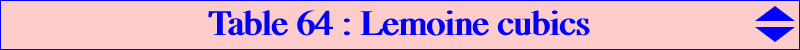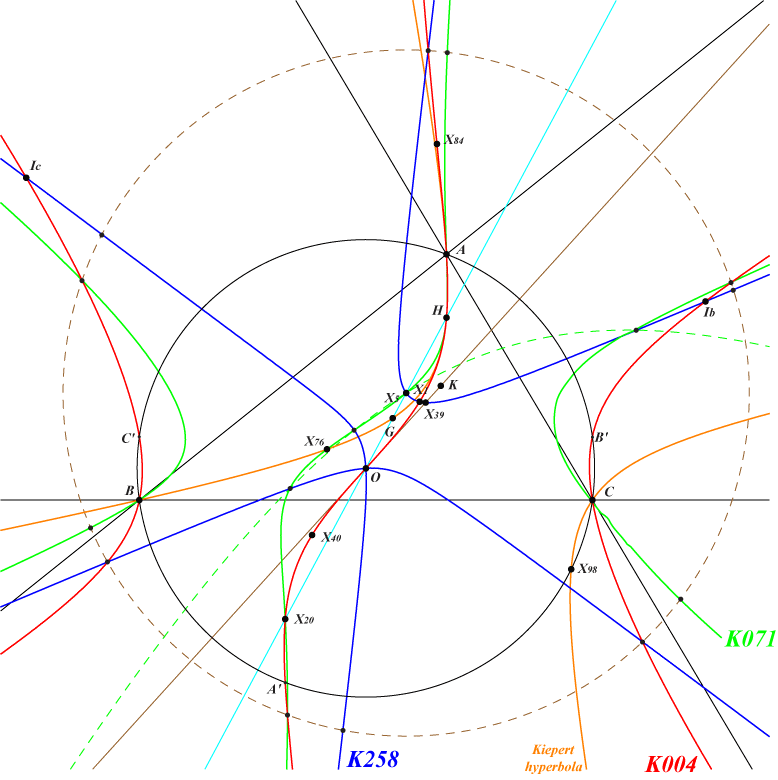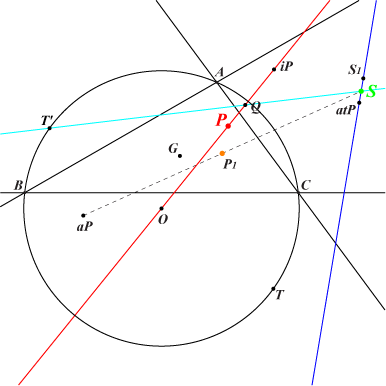This page is intended to summarize and complete the paper : The Lemoine Cubic and its Generalizations, Forum Geometricorum, vol.2 (2002) p.47-63. Let P be a point. Recall that the Lemoine generalized cubic K(P) is a nodal circum-cubic passing through X(4), with node P and perpendicular nodal tangents except the case P = X(4) since it decomposes into the altitudes of ABC. When P lies on the line at infinity, K(P) decomposes into the line at infinity and the rectangular circum-hyperbola passing through P. In all other cases, these nodal tangents are the parallels at P to the hyperbola above. Properties of K(P) (1). K(P) passes through the vertices Pa, Pb, Pc of the pedal triangle of P. (2). K(P) meets the line at infinity at the same points as pK(X6, aP) where aP is the anticomplement of P. (3). K(P) meets the circumcircle (O) at the same points as pK(X6, S) where S is the point with first barycentric : a^2 [ (c^2 (a^2-c^2) q^2 + b^2 (a^2-b^2) r^2 + b^2 c^2 (p^2+2 p q+2 p r-2 q r)) ]. See below for further properties of the mapping P –> S. (4). K(P) passes through the barycentric product Q = P x P⊥ where P⊥ is the orthocorrespondent of P. See the related K934. (5). K(P) meets the altitudes of ABC again at Ha, Hb, Hc on the circle with diameter HP. In other words, Ha, Hb, Hc are the projections of P on the altitudes of ABC. The triangles PaPbPc and HaHbHc are perspective at Q. *** Special cubics K(P) (1). According to property (1) above, K(P) is a psK if and only if P lies on the Darboux cubic K004, in which case its pseudo-pivot lies on the Lucas cubic K007. The pseudo-pole and the pseudo-isopivot respectively lie on two complicated sextics. That corresponding to the pseudo-pole passes through X(20), X(25), X(56), X(184), X(198), X(393). (2). K(P) is a K0 if and only if P lies on the Kiepert hyperbola. It follows that K(P) cannot be a pK unless it decomposes into the altitudes of ABC. (3). K(P) is a K+ if and only if P lies on K071. a cubic passing through X(i) for i = 4, 5, 20, 76, 5562, 15318. (4). K(P) is a cK if and only if P lies on the line at infinity (giving decomposed cubics) or on the circumcircle (O). In this latter case, the root lies on K010 since it is the trilinear pole of the Simson line of P. The only cK0 is then K1325 = K(X98). (5). K(P) is a spK(P', Q') for some points P', Q' if and only if P lies on K258 in which case P' = aP lies on K028, Q' is the midpoint of aP, S on a complicated sextic and K(P) passes through S. K258 passes through X(i) for i = 1, 3, 5, 39, 2140, 14823, 15345, 47055, 51989, 51991, 51999, 52005, 52006, 52010. (6). According to property (2) above, K(P) is a K60 if and only if aP = X(3) hence P = X(5) giving the cubic K054. ***Isogonal transform K(P)* of K(P) K(P)* is obviously also a nodal circum-cubic passing through X(3), with node P*. The nodal tangents are perpendicular if and only if P* and then P lie on the McCay cubic K003. K(P) and K(P)* generate a pencil of circum-cubics which is stable under isogonal conjugation. This pencil contains two self-isogonal cubics, namely : • pK1 = pK(X6, P1) where the pivot P1 is the midpoint Q' of aP-S as above. The first barycentric coordinate of P1 is : a^2 [c^2 SC q^2 + b^2 SB r^2 + b^2c^2 p(q+r) ]. • nK1, an isogonal focal nK with root R1, singular focus on (O). The first barycentric coordinate of R1 is : a^2 [ c^2 SC q^2 - b^2 SB r^2 + b^2c^2 p(q-r) ]. *** The table below gathers the catalogued cubics of CTC. The cubics psK and spK are described in Pseudo-Pivotal Cubics and Poristic Triangles and CL055 respectively. When the cubics pK1, nK1 are unlisted, the pivot P1 or the root R1 is given.P K(P) K(P)* remarks K(P) / K(P)* pK(X6,aP) or aP pK(X6,S) or S Q pK1 nK1 X(3) K009 K028 psK, spK / psK, spK , stelloid K006 K003 X(1147) K005 K187 X(20) K041 psK, K+ / psK X(3146) X(12279) X(27082) X(185) ? X(5) K054 spK, stelloid / K003 X(143) X(143) X(12006) ? X(6) K055 K657 K169 X(22253) X(19136) X(15048) X(3800) X(76) K056 K+ / K410 ? ? ? ? X(2) K295 K297 K002 X(5640) X(1992) X(373) K019 X(1) K360 K259 psK, spK / psK, spK K692 X(145) X(56) note 1 K086 X(40) K926 K654 psK / psK X(692) K004 ? note 1 X(8834) X(98) K1325 cK0 / X(147) X(14510) X(47388) ? ?note 1 : pK1 is the union of the internal bisectors of ABC.The mapping f : P → S Here is a list of ETC pairs {P,S} : {1,145}, {2,5640}, {3,3}, {4,5889}, {5,143}, {6,22253}, {20,12279}, {30,5663}, {40,20}, {74,14508}, {98,14510}, {99,14509}, {100,14513}, {103,14512}, {104,14511}, {110,14480}, {111,14515}, {511,2782}, {512,2782}, {513,952}, {514,2808}, {515,2818}, {516,2808}, {517,952}, {522,2818}, {523,5663}, {1296,14514}, {1350,14532}, {2574,30}, {2575,30}, {3307,517}, {3308,517}, {3413,511}, {3414,511}, {5373,2}, {12518,167}, {12523,1}. • f is a quadratic mapping with no singular point and seven fixed points namely the circumcenter O = X(3), the vertices of the CircumTangential triangle and the infinite points of the McCay cubic K003. • f globally fixes the line at infinity. The infinite points of two perpendicular directions are transformed into the same point which is the infinite point of the line passing through the isogonal conjugates of the two initial points. This latter line is actually the isogonal transform of the rectangular circum-hyperbola passing through the two initial points. See red pairs above. • f maps the circumcircle (O) of ABC onto aH3, the anticomplement of the Steiner deltoid. See blue pairs above. • f maps the reflection SRH in X(3) of the Stammler hyperbola onto the Euler line. See preamble before X(33537) in ETC. SRH passes through the points X(i) for i = 3, 40, 64, 1350, 2574, 2575, 5373, 5646, 9914, 10117, 10620, 11472, 12163, 12301, 12302, 12307, 14926, 15622, 16010, 19376, 22549, 32345, 33535, 33536, 33537, 33538, 33539, 33540, 33541, 33542, 33543. Hence, for any P on SRH, the cubic K(P) meets (O) at the same points as a cubic of the Euler pencil. See Table 27. For instance, K926 = K(X40) meets (O) at the same points as K004 = pK(X6, X20). • f transforms any (finite) line (L) into a parabola (P). When (L) passes through O, (P) also passes through O with tangent (L). The focus F lies on (O) and the directrix is the tangent to (O) at the reflection of F in (L). Construction of S :For P different from O, not lying on the line at infinity, • T is the isogonal conjugate of the infinite point of the line OP and T' is its antipode on (O), • iP is the inverse of P in (O) and Q is the harmonic conjugate of O in P, iP, • atP is the anticomplement of the isotomic conjugate of P, • let Y be the cevapoint of X(6) and the barycentric square of P and let Z be the O-isoconjugate of Y, then S1 is the anticomplement of Z. S is the intersection of the lines Q, T' and atP, S1.Now, if P is a finite point and S = f(P) is its homologue, there are three other points whose image under f is also S. These are the common points (apart P) of three rectangular hyperbolas centered at the vertices of the circumcevian triangle of the isogonal conjugate gS of S. These hyperbolas define an isogonal conjugation with respect to the diagonal triangle of the quadrilateral formed by P and the three points. The vertices Q1, Q2, Q3 of this diagonal triangle are precisely the common points (apart A, B, C) of (O), K(P) and pK(X6, S). It follows that P and the three points are the in/excenters of triangle Q1Q2Q3. When P = O, we have S = O, K(P) = K009, pK(X6, S) = K003 and Q1Q2Q3 is the CircumTangential triangle. When P = X(5373), we have S = G, K(P) is unlisted, pK(X6, S) = K002 and Q1Q2Q3 is the Thomson triangle whose incenter is X(5373).